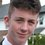# Symmetric Inequality Problem

I am struggling with understanding https://nrich.maths.org/251.

To restate the problem,

If $$x$$, $$y$$ and $$z$$ are real numbers such that: $$\begin{cases}x+y+z=5 \\ xy+yz+zx=3\end{cases}$$ , What is the largest value that any one of these numbers can have?

In particular, I do not understand the first solution given, and while the second I am getting a grip with (creates a quadratic uses the discriminant inequality since $x$, $y$ and $z$ are real numbers), would like to ask whether any classical inequalities can be used here, as I would be personally more satisfied with this.

The problem I had with applying inequalities I knew was that $x$, $y$ and $z$ could be any real numbers, not just positive.

Any help/discussion would be much appreciated!Note by Arthur Conmy
3 years, 4 months ago

This discussion board is a place to discuss our Daily Challenges and the math and science related to those challenges. Explanations are more than just a solution — they should explain the steps and thinking strategies that you used to obtain the solution. Comments should further the discussion of math and science.

When posting on Brilliant:

• Use the emojis to react to an explanation, whether you're congratulating a job well done , or just really confused .
• Ask specific questions about the challenge or the steps in somebody's explanation. Well-posed questions can add a lot to the discussion, but posting "I don't understand!" doesn't help anyone.
• Try to contribute something new to the discussion, whether it is an extension, generalization or other idea related to the challenge.

MarkdownAppears as
*italics* or _italics_ italics
**bold** or __bold__ bold
- bulleted- list
• bulleted
• list
1. numbered2. list
1. numbered
2. list
Note: you must add a full line of space before and after lists for them to show up correctly
paragraph 1paragraph 2

paragraph 1

paragraph 2

[example link](https://brilliant.org)example link
> This is a quote
This is a quote
    # I indented these lines
# 4 spaces, and now they show
# up as a code block.

print "hello world"
# I indented these lines
# 4 spaces, and now they show
# up as a code block.

print "hello world"
MathAppears as
Remember to wrap math in $$ ... $$ or $ ... $ to ensure proper formatting.
2 \times 3 $2 \times 3$
2^{34} $2^{34}$
a_{i-1} $a_{i-1}$
\frac{2}{3} $\frac{2}{3}$
\sqrt{2} $\sqrt{2}$
\sum_{i=1}^3 $\sum_{i=1}^3$
\sin \theta $\sin \theta$
\boxed{123} $\boxed{123}$

Sort by:

The link u have referred is not opening, it says the page not found.However, u can see my solution to this problem : https://brilliant.org/problems/almost-vietas/#!/solution-comments/171217/

- 3 years, 4 months ago

Fixed btw

- 3 years, 4 months ago

yup

- 3 years, 4 months ago

- 3 years, 4 months ago

actually u have put a dot by mistake in front of 251

- 3 years, 4 months ago

okay ! great

- 3 years, 4 months ago

Hey buddy is the answer $\frac{13}{2}$?

- 3 years, 4 months ago

i m getting $\frac{13}{3}$ as maximum value and -1 as minimum value

- 3 years, 4 months ago

Yep same here! What was your method?

- 3 years, 4 months ago

but u said u are getting $\frac{13}{2}$ . I used the same method which i referred to in the link i gave.

- 3 years, 4 months ago

Oops! Sorry I am getting $\frac{13}{3}$. I actually did not check the link out. Let me check it now

- 3 years, 4 months ago

- 3 years, 4 months ago

Cauchy Schwarz inequality

- 3 years, 4 months ago

same method as mine then? or in a different way?

- 3 years, 4 months ago